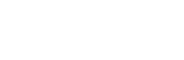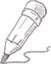#2022-06-02   阅读量: 110

Mysql

## 一道根据行内容创建新列的SQL题,要求对学生成绩进行等级判断并输出给定的表格示例

create table stuscore

(sname varchar(10) ,course varchar(10) ,score int);

insert into stuscore values

('ZS','语文',90),

('ZS','数学',89),

('李四','语文',86),

('李四','英语',84),

('李四','数学',75),

('ZS','英语',56);

#要求对学生成绩进行等级判断,大于等于80,优秀;大于等于60,及格;小于60,不及格。并输出下面的给定表格示例:

# 姓名 语文 数学 英语

# ZS 优秀 优秀 不及格

# 李四 优秀 及格 优秀

```use cda;
create table stuscore
(sname varchar(10) ,course varchar(10) ,score int);
insert into stuscore values
('ZS','语文',90),
('ZS','数学',89),
('李四','语文',86),
('李四','英语',84),
('李四','数学',75),
('ZS','英语',56);

#1,要求对学生成绩进行等级判断,大于等于80,优秀;大于等于60,及格;小于60,不及格。
#并输出下面的给定表格示例:
# 姓名 语文 数学 英语
# ZS   优秀 优秀 不及格
# 李四 优秀 及格 优秀

select  sname,
case
when 语文成绩>=80 then "优秀"
when 语文成绩>=60 and 语文成绩=80 then "优秀"
when 数学成绩>=60 and 数学成绩=80 then "优秀"
when 英语成绩>=60 and 英语成绩<80 then "及格"
else "不及格"
end as 英语
from
(
select sname,
sum(if(course='语文',score,null)) 语文成绩,
sum(if(course='数学',score,null)) 数学成绩,
sum(if(course='英语',score,null)) 英语成绩
from stuscore
group by sname) t;

select sname,
sum(if(course='语文',score,null)) 语文成绩,
sum(if(course='数学',score,null)) 数学成绩,
sum(if(course='英语',score,null)) 英语成绩
from stuscore
group by sname;```

20.2572 2 0 关注作者 收藏

## 评论(0)